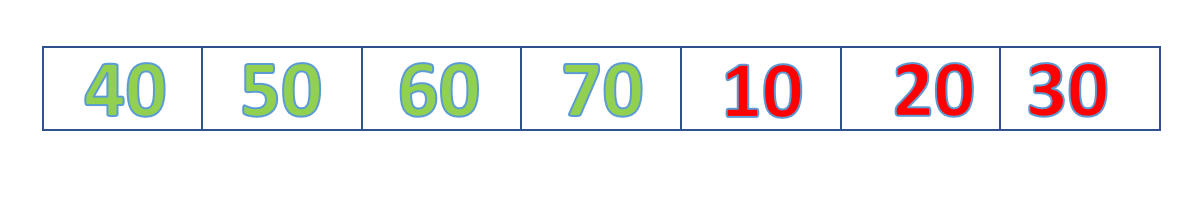Related Articles
Java Program to Rotate Elements of the List
• Difficulty Level : Medium
• Last Updated : 28 Dec, 2020

List is an ordered collection of objects in which duplicate values can be stored. Since List preserves the insertion order, it allows positional access and insertion of elements. In this article, we are going to see how to rotate elements of a list. Let’s consider the following list.ORIGINAL LIST BEFORE ROTATION

There are two types of rotations in a list. They are right rotation and left rotation. After Four Right Rotations the list becomes as shown below:LIST AFTER  ROTATING FOUR POSITIONS

Method 1: (Without Using in-built methods)

Working For Right Rotation

• First store the last element of the list in a temp variable.
• Move the elements in one position towards the right.
• Now change the first element value of the list with value in a temp variable.
• Update the value to a temp variable to the new last element.
• Repeat the above steps required a number of rotations.Example:

## Java

 `// Java Program to Rotate Elements of the List``import` `java.io.*;``import` `java.util.*;``class` `GFG {``   ` `    ``public` `static` `void` `main(String[] args)``    ``{``        ``// creating ArrayList``        ``List my_list = ``new` `ArrayList<>();``        ``my_list.add(``10``);``        ``my_list.add(``20``);``        ``my_list.add(``30``);``        ``my_list.add(``40``);``        ``my_list.add(``50``);``        ``my_list.add(``60``);``        ``my_list.add(``70``);`` ` `        ``// Printing list before rotation``        ``System.out.println(``            ``"List Before Rotation : "``            ``+ Arrays.toString(my_list.toArray()));`` ` `        ``// Loop according to the number of rotations``        ``for` `(``int` `i = ``0``; i < ``4``; i++) {``           ` `            ``// storing the last element in the list``            ``int` `temp = my_list.get(``6``);``           ` `            ``// traverse the list and move elements to right``            ``for` `(``int` `j = ``6``; j > ``0``; j--) {``                ``my_list.set(j, my_list.get(j - ``1``));``            ``}``            ``my_list.set(``0``, temp);``        ``}`` ` `        ``// Printing list after rotation``        ``System.out.println(``            ``"List After Rotation :  "``            ``+ Arrays.toString(my_list.toArray()));``    ``}``}`
Output
```List Before Rotation : [10, 20, 30, 40, 50, 60, 70]
List After Rotation :  [40, 50, 60, 70, 10, 20, 30]```

After Four Left Rotations the list becomes as shown below:LIST AFTER ROTATING FOUR POSITIONS

Working For Left Rotation

• First store the first element of the list in a temp variable.
• Move the elements in one position towards the left.
• Now change the last element value of the list with the value in the temp variable.
• Update the value to a temp variable to the new first element.
• Repeat the above steps required a number of rotations.Example

## Java

 `// Java Program to Rotate Elements of the List`` ` `import` `java.io.*;``import` `java.util.*;``class` `GFG {``    ``public` `static` `void` `main(String[] args)``    ``{``        ``// creating array list``        ``List my_list = ``new` `ArrayList<>();``        ``my_list.add(``10``);``        ``my_list.add(``20``);``        ``my_list.add(``30``);``        ``my_list.add(``40``);``        ``my_list.add(``50``);``        ``my_list.add(``60``);``        ``my_list.add(``70``);`` ` `        ``// Printing list before rotation``        ``System.out.println(``            ``"List Before Rotation : "``            ``+ Arrays.toString(my_list.toArray()));`` ` `        ``// Loop according to the number of rotations``        ``for` `(``int` `i = ``0``; i < ``4``; i++) {``            ``// storing the first element in the list``            ``int` `temp = my_list.get(``0``);``            ``// traverse the list and move elements to left``            ``for` `(``int` `j = ``0``; j < ``6``; j++) {``                ``my_list.set(j, my_list.get(j + ``1``));``            ``}``            ``my_list.set(``6``, temp);``        ``}`` ` `        ``// Printing list after rotation``        ``System.out.println(``            ``"List After Rotation :  "``            ``+ Arrays.toString(my_list.toArray()));``    ``}``}`
Output
```List Before Rotation : [10, 20, 30, 40, 50, 60, 70]
List After Rotation :  [50, 60, 70, 10, 20, 30, 40]```

Method 2: (Rotation Using Collections.rotate(list, distance) method)

Both left and right rotations can be performed directly using Java Collections.

Syntax

Collections.rotate(list_name , distance)

Parameters:

• list_name: name of the list.
• distance: Distance is the number of elements that we have to rotate.

Returns: It returns a rotated list.

Note: Negative Distance gives Left Rotation while Positive gives Right Rotation.

Example: Using Positive distance to get the Right Rotation.

## Java

 `// Java Program to Rotate Elements of the List`` ` `import` `java.io.*;``import` `java.util.*;``class` `GFG {``    ``public` `static` `void` `main(String[] args)``    ``{``        ``// creating array list``        ``List my_list = ``new` `ArrayList<>();``        ``my_list.add(``10``);``        ``my_list.add(``20``);``        ``my_list.add(``30``);``        ``my_list.add(``40``);``        ``my_list.add(``50``);``        ``my_list.add(``60``);``        ``my_list.add(``70``);`` ` `        ``// Printing list before rotation``        ``System.out.println(``            ``"List Before Rotation : "``            ``+ Arrays.toString(my_list.toArray()));`` ` `        ``// Rotating the list at distance 4`` ` `        ``Collections.rotate(my_list, ``4``);`` ` `        ``// Printing list after rotation``        ``System.out.println(``            ``"List After Rotation :  "``            ``+ Arrays.toString(my_list.toArray()));``    ``}``}`
Output
```List Before Rotation : [10, 20, 30, 40, 50, 60, 70]
List After Rotation :  [40, 50, 60, 70, 10, 20, 30]```

Example: Using Negative distance to get the Left Rotation.

## Java

 `// Java Program to Rotate Elements of the List`` ` `import` `java.io.*;``import` `java.util.*;``class` `GFG {``    ``public` `static` `void` `main(String[] args)``    ``{``        ``// creating array list``        ``List my_list = ``new` `ArrayList<>();``        ``my_list.add(``10``);``        ``my_list.add(``20``);``        ``my_list.add(``30``);``        ``my_list.add(``40``);``        ``my_list.add(``50``);``        ``my_list.add(``60``);``        ``my_list.add(``70``);`` ` `        ``// Printing list before rotation``        ``System.out.println(``            ``"List Before Rotation : "``            ``+ Arrays.toString(my_list.toArray()));`` ` `        ``// Rotating the list at distance -3``        ``Collections.rotate(my_list, -``4``);`` ` `        ``// Printing list after rotation``        ``System.out.println(``            ``"List After Rotation :  "``            ``+ Arrays.toString(my_list.toArray()));``    ``}``}`
Output
```List Before Rotation : [10, 20, 30, 40, 50, 60, 70]
List After Rotation :  [50, 60, 70, 10, 20, 30, 40]```

Attention reader! Don’t stop learning now. Get hold of all the important Java Foundation and Collections concepts with the Fundamentals of Java and Java Collections Course at a student-friendly price and become industry ready. To complete your preparation from learning a language to DS Algo and many more,  please refer Complete Interview Preparation Course.

My Personal Notes arrow_drop_up